### IMO Shortlist 2013 problem G5

Kvaliteta:
Avg: 0,0
Težina:
Avg: 8,0
Let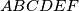$ABCDEF$ be a convex hexagon with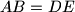$AB = DE$,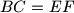$BC = EF$,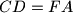$CD = FA$, and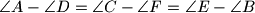$\angle A - \angle D = \angle C - \angle F = \angle E - \angle B$. Prove that the diagonals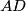$AD$,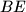$BE$, and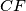$CF$ are concurrent.
Izvor: Ukraine